# 基于Picard迭代的PN×PN-2谱元法求解定常不可压缩Navier-Stokes方程*

(1. 重庆交通大学 重庆西南水运工程科学研究所， 重庆 400016;2. 重庆交通大学 内河航道整治技术交通行业重点实验室， 重庆 400074;3. 重庆西科水运工程咨询中心， 重庆 400016;4. 重庆大学 航空航天学院， 重庆 400044)

## 1 数 值 方 法

### 1.1 PN×PN-2谱元法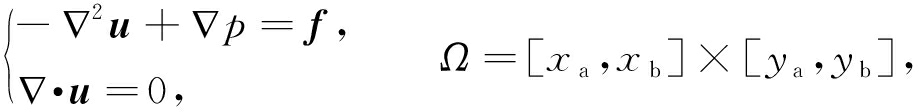(1)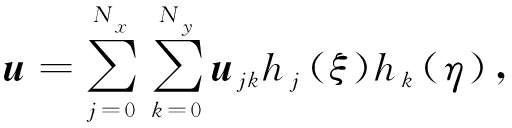(2)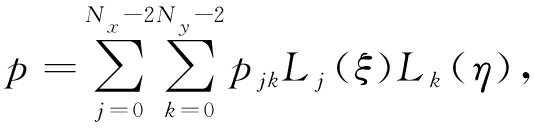(3)

wx=wy=hm(ξ)hn(η), 0≤mNx, 0≤nNy,

(4)

q=Lm(ξ)Ln(η), 0≤mNx-2, 0≤nNy-2.

(5)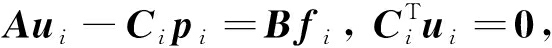(6)

Ajkmn=Ajm·Bkn+Bjm·Akn,

(7a)

Bjkmn=Bjm·Bkn,

(7b)

(C1)jkmn=Cjm·Fkn,

(7c)

(C2)jkmn=Fjm·Ckn,

(7d)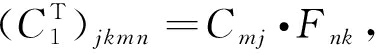(7e)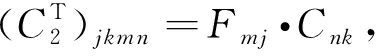(7f)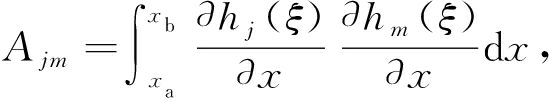(7g)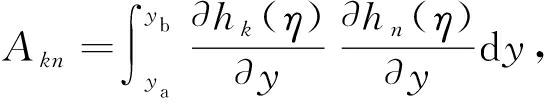(7h)

Bjm=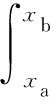hj(ξ)hm(ξ)dx

(7i)

Bkn=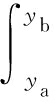hk(η)hn(η)dy

(7j)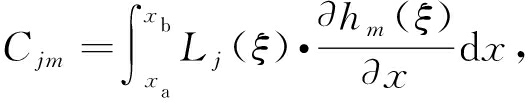(7k)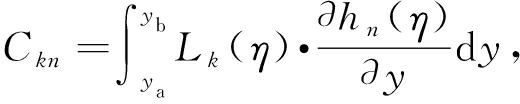(7l)

Fjm=Lj(ξ)hm(ξ)dx

(7m)

Fkn=Lk(η)hn(η)dy.

(7n)

### 1.2 Picard迭代法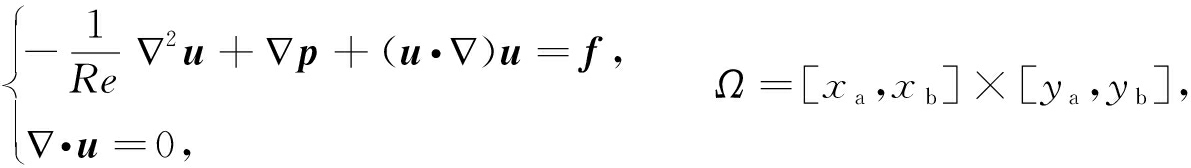(8)

-ν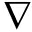2uk+1+pk+1+(uk·)uk+1=f,

(9)·uk+1=0,

(10)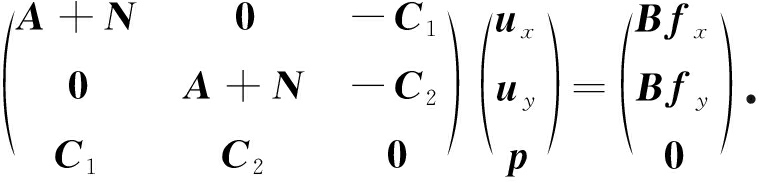(11)

## 2 数值算例与结果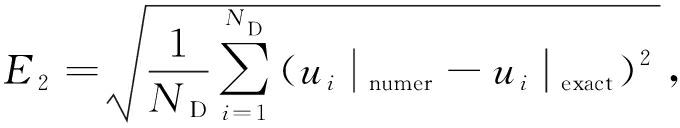(12)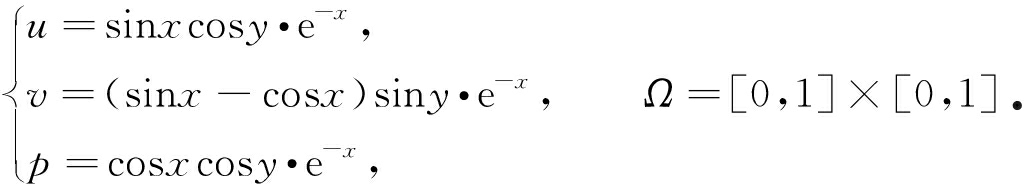(13)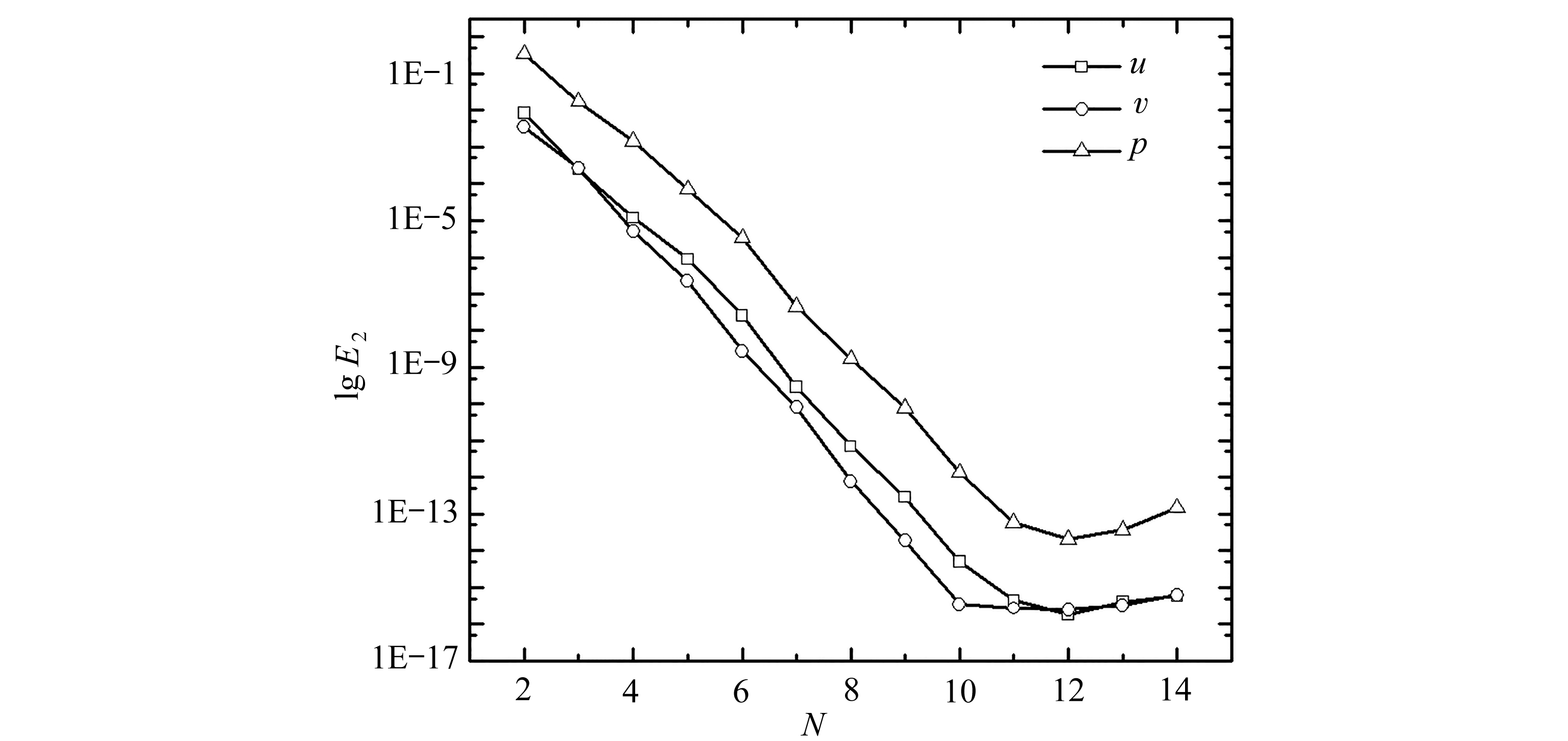Fig. 1 Dependency of the 2-norm error on discrete order N for example 1(a) PN×PN谱元法 (b) 本文方法 (c) 精确压力场
(a) The PN×PN spectral element method (b) This paper method (c) The exact pressure field

Fig. 2 The pressure fields obtained with the PN×PN spectral element method and the PN×PN-2
spectral element method, along with the exact pressure field for example 1

Kovasznay流动是由Kovasznay给出的一组NS方程分析解: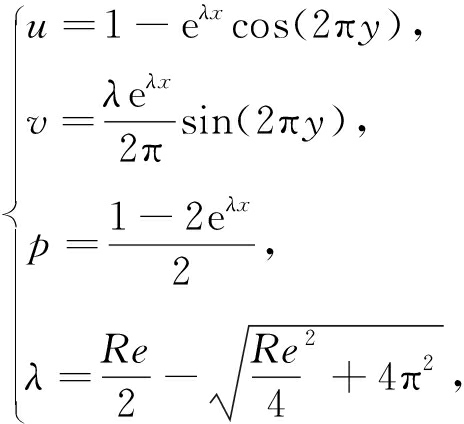(14)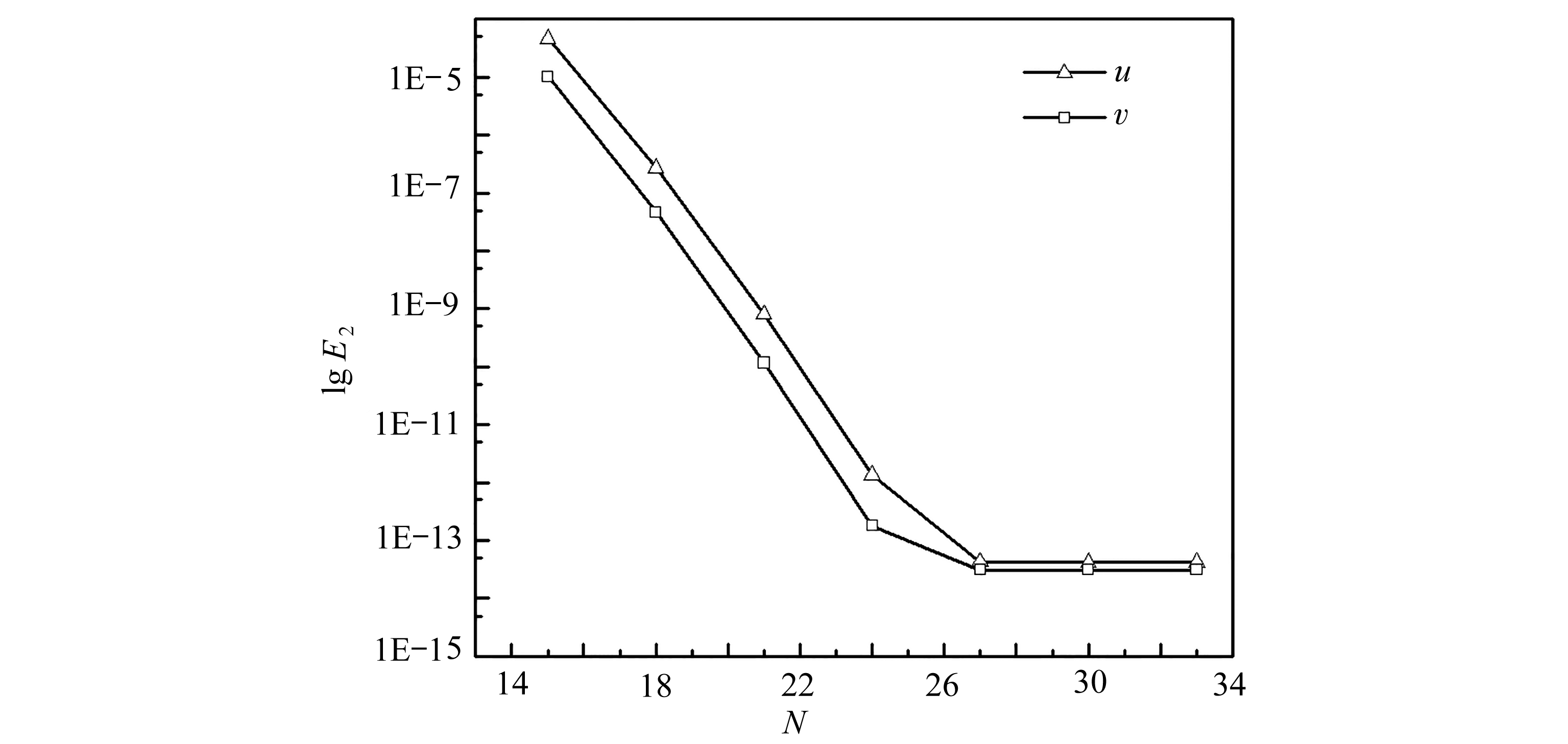Fig. 3 Dependency of the 2-norm error on the discrete orders for the Kovasznay flow

Table 1 The number of iteration steps under different discrete orders for simulation for the Kovasznay flow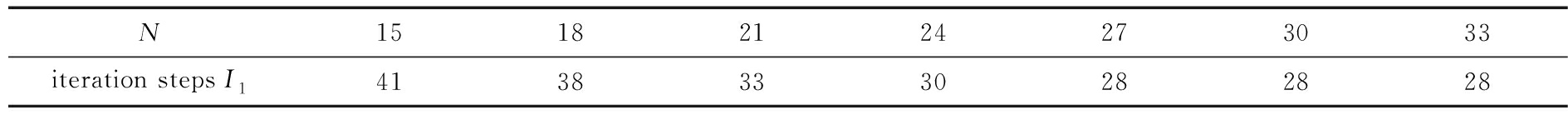N15182124273033iteration steps I141383330282828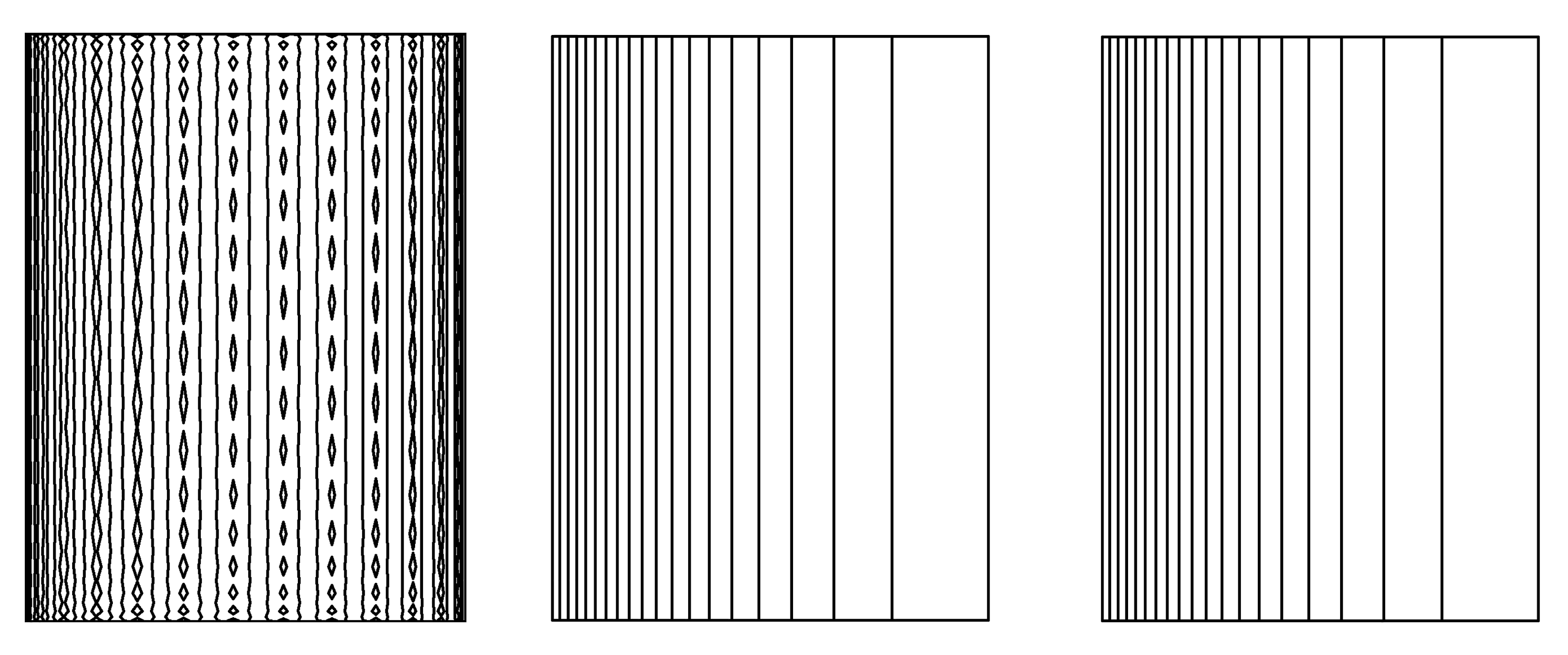(a) PN×PN谱元法 (b) 本文方法 (c) 精确压力场
(a) The PN×PN spectral element method (b) This paper method (c) The exact pressure field

Fig. 4 The pressure fields obtained with the PN×PN spectral element method and the PN×PN-2
spectral element method, along with the exact pressure field example 2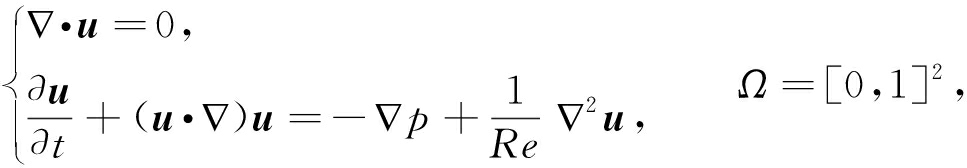(15)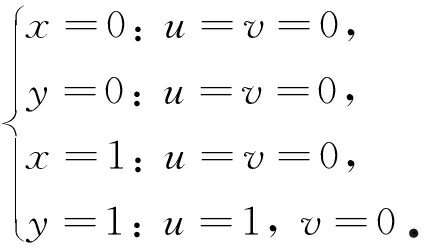(16)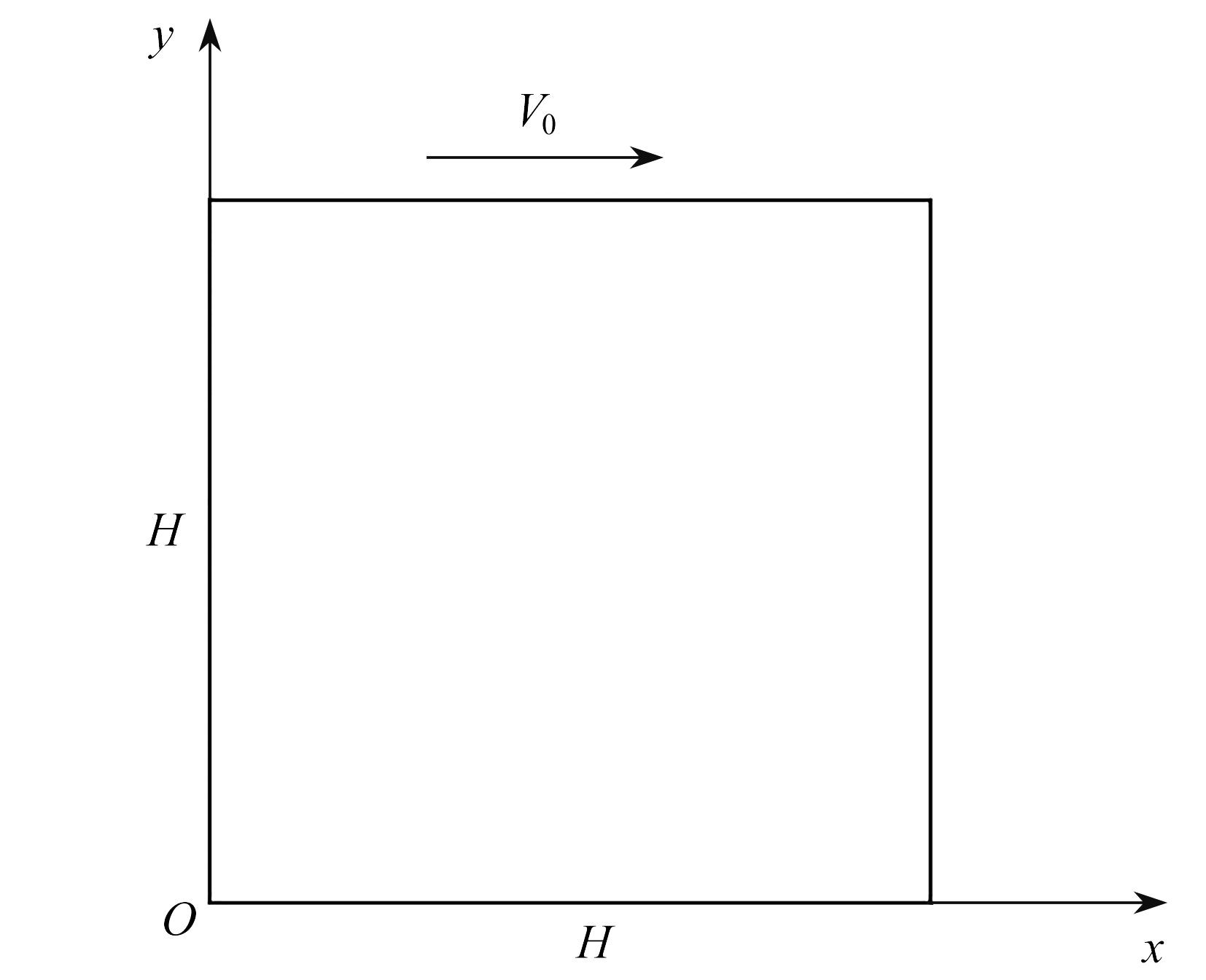Fig. 5 The configuration of the lid-driven cavity flow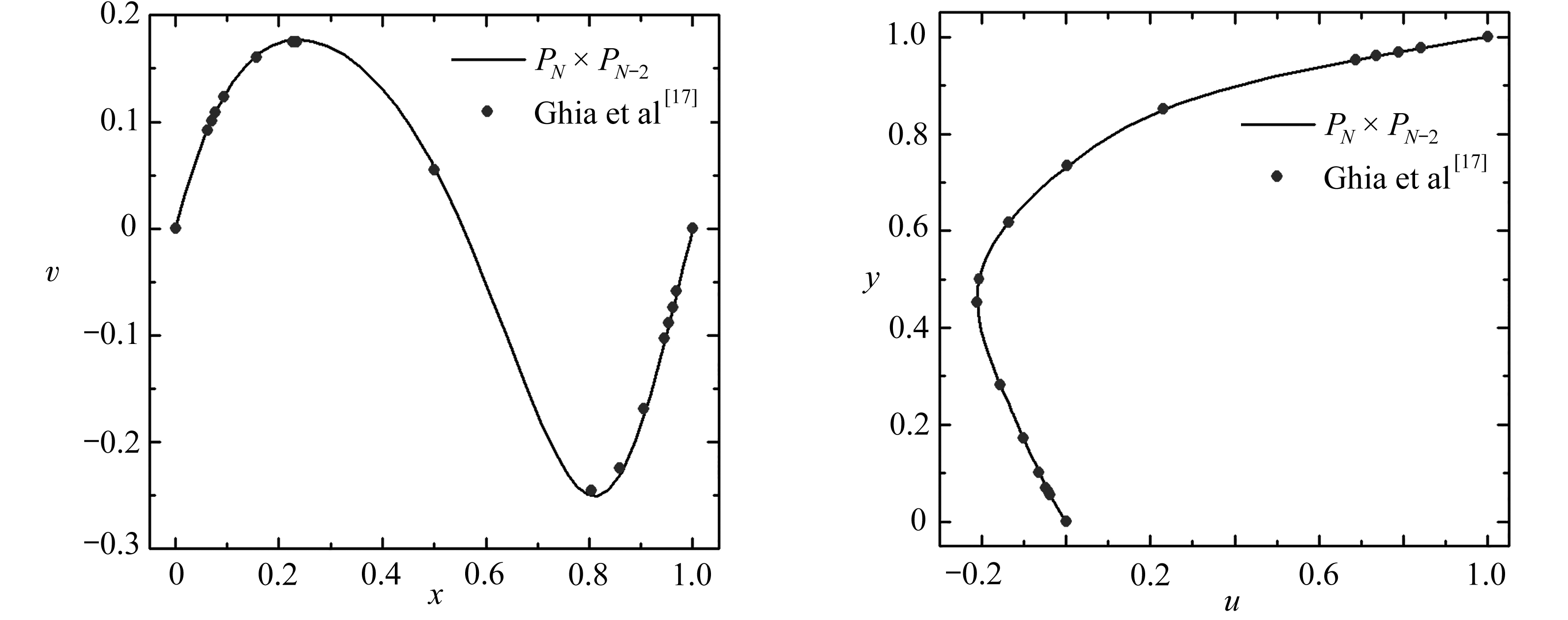(a) Re=100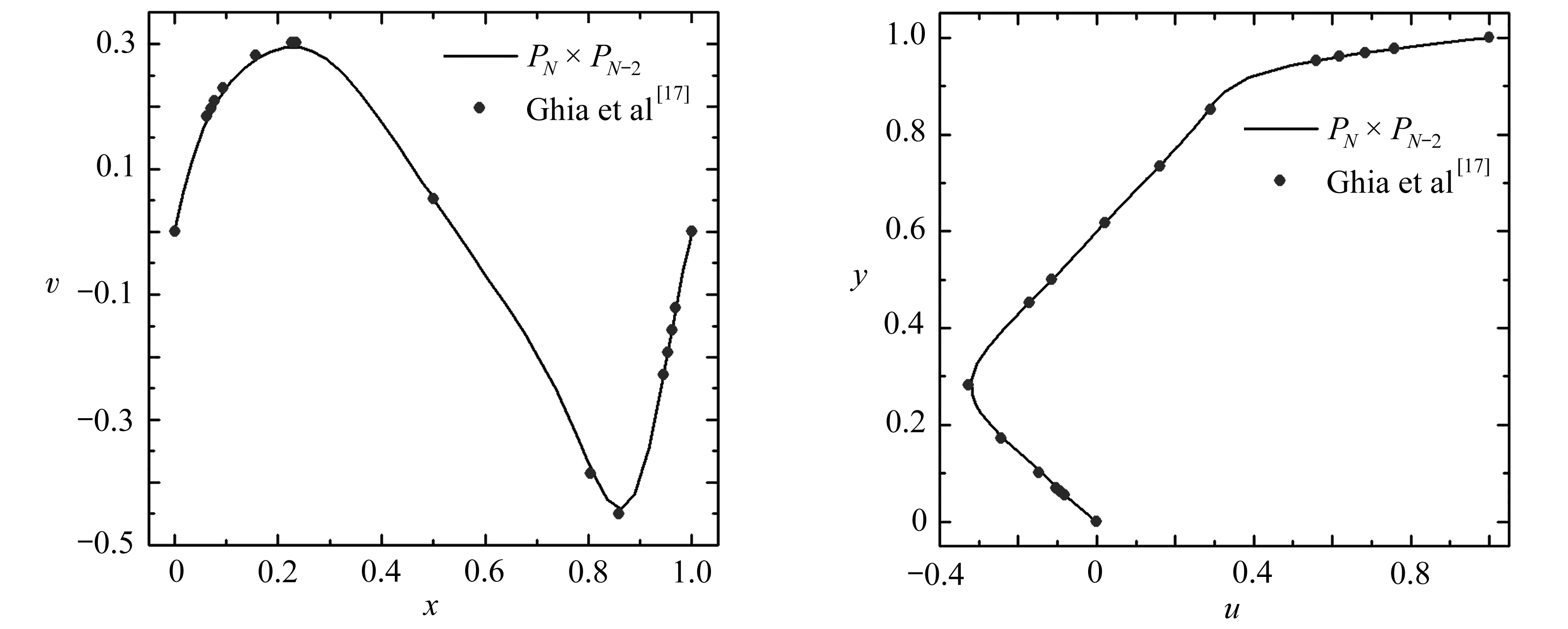(b) Re=400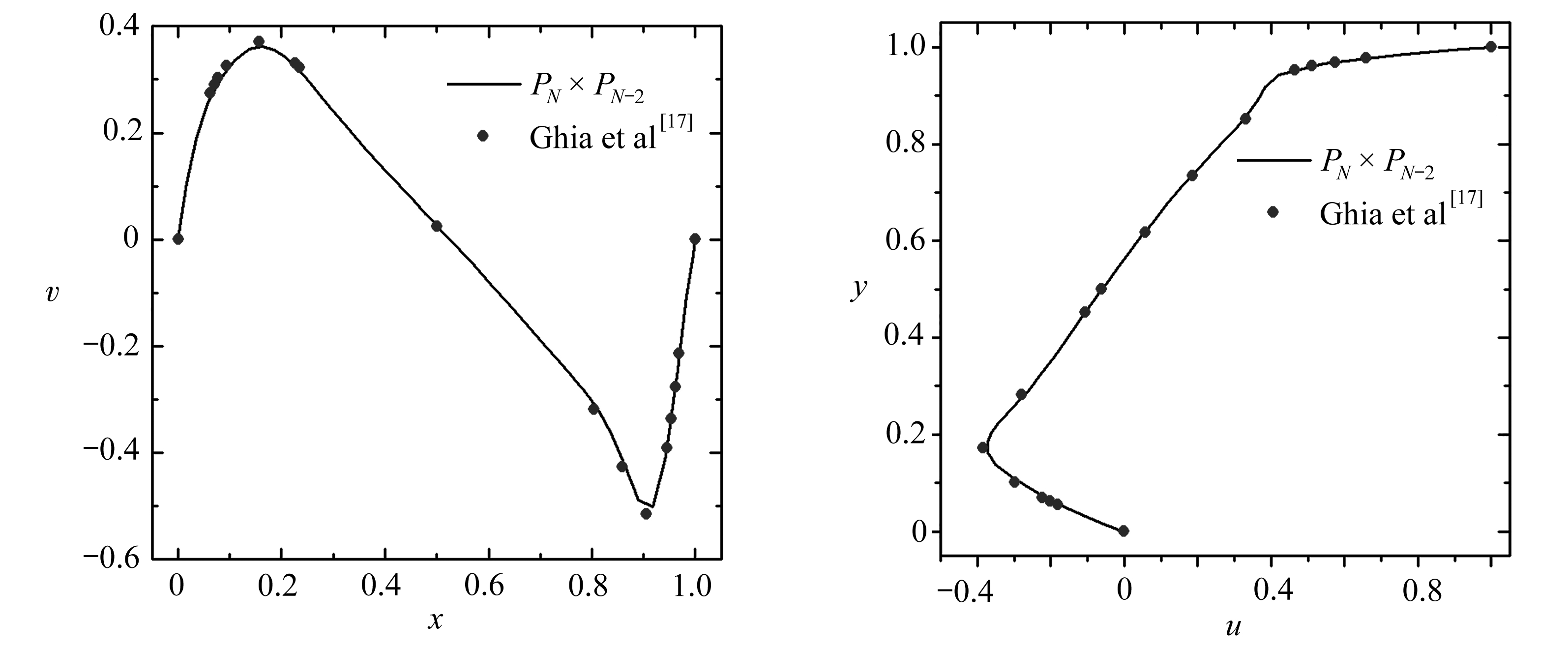(c) Re=1 000

Fig. 6 Velocities along the center line for the lid-driven cavity flow with different Re values: y=0.5(left) and x=0.5(right)

## 3 结 论

 AUTERI F, GUERMOND J L, PAROLINI N. Role of the LBB condition in weak spectral projection methods[J]. Journal of Computational Physics, 2001, 174(1): 405-420.

 SCHUMACK M R, SCHULTZ W W, BOYD J P. Spectral method solution of the Stokes equations on nonstaggered grids[J]. Journal of Computational Physics, 1990, 89(2): 30-58.

 DEBLOIS B M. Linearizing convection terms in the Navier-Stokes equations[J]. Computer Methods in Applied Mechanics and Engineering, 1997, 143(3/4): 289-297.

 REHMAN M U, VUIK C, SEGAL G. Numerical solution techniques for the steady incompressible Navier-Stokes problem[C]//Proceedings of the World Congress on Engineering. London, 2008: 844-849.

 苏铭德, 陈霜立. 定常不可压缩粘性流体流动Navier-Stokes方程的推进迭代法[J]. 计算物理, 1989, 6: 321-334.(SU Mingde, CHEN Shuangli. Solution of the N-S equation of the steady incompressible viscous flow with marching-iterative method[J]. Chinese Journal of Computational Physics, 1989, 6: 321-334.(in Chinese))

 CASARIN M A. Schwarz preconditioners for the spectral element discretization of the steady Stokes and Navier-Stokes equations[J]. Numerische Mathematik, 2001, 89: 307-339.

 PONTAZA J P, REDDY J N. Spectral/hp least-squares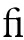nite element formulation for the Navier-Stokes equations[J]. Journal of Computational Physics, 2003, 190: 523-549.

 KNOLL D A, KEYES D. Jacobian-free Newton-Krylov methods: a survey of approaches and applications[J]. Journal of Computational Physics, 2004, 193: 357-397.

 马东军, 柳阳, 孙德军, 等. 高阶谱元区域分解算法求解定常方腔驱动流[J]. 计算力学学报, 2006, 23(6): 668-673.(MA Dongjun, LIU Yang, SUN Dejun, et al. Spectral element method with a domain decomposition Stokes solver for steady cavity driven flow[J]. Chinese Journal of Computational Mechanics, 2006, 23(6): 668-673.(in Chinese))

 ZHANG W, ZHANG C H, XI G. An explicit Chebyshev pseudospectral multigrid method for incompressible Navier-Stokes equations[J]. Computers & Fluids, 2010, 39(1): 178-188.

 章争荣, 张湘伟. 二维定常不可压缩粘性流动N-S方程的数值流形方法[J]. 计算力学学报, 2010, 27(3): 415-421.(ZHANG Zhengrong, ZHANG Xiangwei. Numerical manifold method for steady incompressible viscous 2D flow Navier-Stokes equtions[J]. Chinese Journal of Computational Mechanics, 2010, 27(3): 415-421.(in Chinese))

 覃燕梅, 冯民富, 罗鲲, 等. Navier-Stokes方程的局部投影稳定化方法[J]. 应用数学和力学, 2010, 31(5): 618-630.(QIN Yanmei, FENG Minfu, LUO Kun, et al. Local projection stabilized finite element method for the Navier-Stokes equations[J]. Applied Mathematics and Mechanics, 2010, 31(5): 618-630.(in Chinese))

 HE Y, ZHANG Y, SHANG Y, et al. Two-level Newton iterative method for the 2D/3D steady Navier-Stokes equations[J]. Numerical Methods for Partial Differential Equations, 2012, 28: 1620-1642.

 MELCHIOR S A, LEGAT V, DOOREN P V, et al. Analysis of preconditioned iterative solvers for incompressible flow problems[J]. International Journal for Numerical Methods in Fluids, 2012, 68: 269-286.

 OZCELIKKALE A, SERT C. Least-squares spectral element solution of incompressible Navier-Stokes equations with adaptive refinement[J]. Journal of Computational Physics, 2012, 231: 3755-3769.

 戴海, 潘文峰. 谱元法求解Helmholtz方程透射特征值问题[J]. 应用数学和力学, 2018, 39(7): 833-840.(DAI Hai, PAN Wenfeng. A spectral element method for transmission eigenvalue problems of the Helmholtz equation[J]. Applied Mathematics and Mechanics, 2018, 39(7): 833-840.(in Chinese))

 GHIA U, GHIA K N, SHIN C T. High-Re solutions for incompressible flow using the Navier-Stokes equations and a multigrid method[J]. Journal of Computational Physics, 1982, 48(3): 387-411.

 AIDUN C K, TRIANTAFILLOPOULOS N G, BENSON J. Global stability of a lid-driven cavity with through-flow: flow visualization studies[J]. Physics of Fluids A: Fluid Dynamics, 1991, 3(9): 2081-2091.

 ALBENSOEDER S, KUHLMANN H C, RATH H J. Three-dimensional centrifugal-flow instabilities in the lid-driven-cavity problem[J]. Physics of Fluids, 2001, 13: 121-135.

 THEOFILIS V. Global linear instability[J]. Annual Review of Fluid Mechanics, 2011, 43: 319-352.

 KOSEFF J R, STREET R L. The lid-driven cavity flow: a synthesis of qualitative and quantitative observations[J]. Journal of Fluids Engineering, 1984, 106(4): 390-398.

 KOSEFF J R, STREET R L, GRESHO P M, et al. A three-dimensional lid-driven cavity flow: experiment and simulation[C]//International Conference on Numerical Methods in Laminar and Turbulent Flow. Seattle, WA, 1983.

# A PN×PN-2 Spectral Element Method Based on the Picard Iteration for Steady Incompressible Navier-Stokes Equations

QIU Zhouhua1,2,3, ZENG Zhong4, LIU Hao1,2,3

(1. Chongqing Southwest Research Institute for Water Transport Engineering, Chongqing Jiaotong University, Chongqing 400016, P.R.China;2. Key Laboratory of Transport Industry of Inland Waterway Regulation EngineeringChongqing Jiaotong University, Chongqing 400074, P.R.China;3. Chongqing Xike Waterway Engineering Consulting Center, Chongqing 400016, P.R.China;4. College of Aerospace Engineering, Chongqing University, Chongqing 400044, P.R.China)

Abstract: A PN×PN-2 spectral element method based on the Picard linearized iteration was presented for the solution of 2D steady incompressible Navier-Stokes equations. Through the Picard iteration, the Navier-Stokes equations were converted to a series of Stokes-type equations to be solved with the PN×PN-2 spectral element method on the non-staggered grid in each iteration step. In order to eliminate the pseudo pressure mode, the pressure discretization is 2 orders lower than the velocity discretization, and the application of non-staggered grids makes the discretization of the equation convenient and avoids the interpolation error. The Stokes flow, the Kovasznay flow and the lid-driven cavity flow were simulated with the present method. The numerical results show that, the error converges with the spectral accuracy. In addition, avoidance of the pressure oscillation phenomenon indicates the accuracy and reliability of the present method.

Key words: steady Navier-Stokes equations; Picard iteration; spectral element method; pseudo pressure mode

(我刊编委曾忠来稿)

DOI：10.21656/1000-0887.410289

(Contributed by ZENG Zhong, M. AMM Editorial Board)

*收稿日期： 2020-09-24； 修订日期：2020-10-12